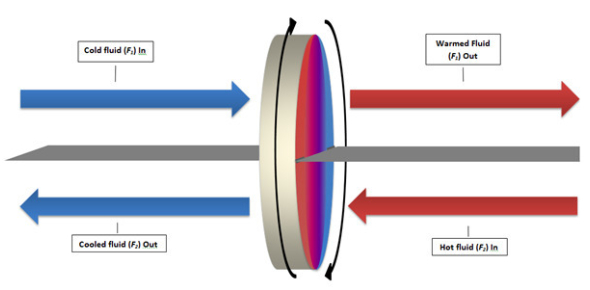# Science Test: Types Of Heat And Their Properties

22 Questions | Attempts: 7170SettingsHeat is the transfer of energy from a hot object to one with lover temperature. Heat occurs in different types, and we got to cover all of them and how they move from one object to another. How well did you understand the topic? Take up this test and see if you may need to get a science tutor. Good luck!

• 1.
What is a tool that is used to measure temperature?
• A.

Scale

• B.

Thermometer

• C.

Conductor

• D.

Friction

• 2.
What kind of material that allows heat to move through it easily?
• A.

Conductor

• B.

Thermometer

• C.

Friction

• D.

Insulator

• 3.
What is aforce that occurs wen one object rubs against another object?
• A.

Thermal energy

• B.

Solar energy

• C.

Friction

• D.

Conduction

• 4.
Which energy is the energy of moving particles in matter?
• A.

Solar energy

• B.

Thermal energy

• C.

Conduction

• D.

Friction

• 5.
What is energy from the sun called?
• A.

Sun Energy

• B.

Sun Burn

• C.

Thermal Energy

• D.

Solar Energy

• 6.
What material does not allow heat to pass through it easily?
• A.

Insulator

• B.

Insulation

• C.

Conductor

• D.

Friction

• 7.
What is the movement of heat within a solid or from one solid to another?
• A.

Transfer of Energy

• B.

Conduction

• C.

Insulation

• D.

Conductor

• 8.
Rubbing your hands together is an example of which type of energy?
• A.

Solar Energy

• B.

Friction

• C.

Thermal

• D.

Conduction

• 9.
Cooking is an example of which type of energy?
• A.

Thermometer

• B.

Solar Energy

• C.

Insulator

• D.

Conduction

• 10.
What is the way heat moves through liquids and gases called?
• A.

Convection

• B.

Conduction

• C.

• D.

Thermal Energy

• 11.
Which of the examples below is an example of Convection?
• A.

• B.

Heating a fish tank

• C.

• D.

Striking a match

• 12.
What is the movement of energy by waves called?
• A.

Thermal Energy

• B.

Conduction

• C.

• D.

Solar Energy

• 13.
Being warmed by fire is an example of which type of energy?
• A.

• B.

When you cool down your hot chocolate by putting cold milk in it.

• C.

Rubbing sticks

• D.

• 14.
What are the 3 kinds of conductors?
• A.

Styrofoam, glass, plastic

• B.

Metal, glass, ceramic

• C.

Glass, wood, liquid

• D.

Wood, plastic, paper

• 15.
What colors reflect solar energy better?
• A.

Light colors, example: snow

• B.

Dark colors: A black shirt

• C.

Neon colors

• D.

Primary colors, the color blue

• 16.
What colors asborb solar energy better?
• A.

Dark colors

• B.

Light colors

• C.

Primary colors

• D.

Neon colors

• 17.
What keeps thermal energy from moving?
• A.

When one item is hot and the other is cold

• B.

When the items are the same temperature

• C.

When one item is glass and the other is plastic

• 18.
What is an example of thermal energy?
• A.

Putting a spoon in hot grits and the spoon gets hot

• B.

• C.

• 19.
Why does the liquid in a thermometer rise on a hot day and drop down on a cold day?
• A.

On a hot day, the particles in the liquid move slow and take up less space. On a cold day the particles in the liquid move fast and take up more space.

• B.

On a hot day, the particles in the liquid move fast and take up more space. On a cold day the particles in the liquid move slowly and take up less space.

• C.

On a hot day, the particles in the liquid move slow and take up less space. On a cold day the particles in the liquid don't move and take no space.

• 20.
What happens when a hot thing touches a cold thing and the heat from the hot thing makes the cold particles speed up?
• A.

Convection

• B.

Conduciton

• C.

• 21.
What happens when heat warm up the air particles around it and the hot air rises and pushes the cold air down?
• A.

• B.

Conduction

• C.

Convection

• 22.
What happens when heat moves around radiation and the heat waves that some from a het source travel to an object and are absorbed by that object?
• A.

• B.

Convection

• C.

Conduction

## Related TopicsBack to top
×

Wait!
Here's an interesting quiz for you.